Two short magnets of equal dipole moments M are fastened perpendicularly at their centres (figure). The magnitude of the magnetic field at a distance d from the centre on the bisector of the right angle is :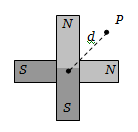1.

2.

3.

4.

Concept Questions :-

Bar magnet
High Yielding Test Series + Question Bank - NEET 2020

Difficulty Level:

Two identical bar magnets with a length 10 cm and weight 50 gm-weight are arranged freely with their like poles facing in a inverted vertical glass tube. The upper magnet hangs in the air above the lower one so that the distance between the nearest pole of the magnet is 3mm. Pole strength of the poles of each magnet will be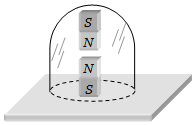(a) 6.64 amp$×$m

(b) 2 amp$×$m

(c) 10.25 amp$×$m

(d) None of these

Concept Questions :-

Analogy between electrostatics and magnetostatics
High Yielding Test Series + Question Bank - NEET 2020

Difficulty Level:

If ${\varphi }_{1}$ and ${\varphi }_{2}$ be the angles of dip observed in two vertical planes at right angles to each other and $\varphi$ be the true angle of dip, then

1. ${\mathrm{cos}}^{2}\varphi ={\mathrm{cos}}^{2}{\varphi }_{1}+{\mathrm{cos}}^{2}{\varphi }_{2}$

2. ${\mathrm{sec}}^{2}\varphi ={\mathrm{sec}}^{2}{\varphi }_{1}+{\mathrm{sec}}^{2}{\varphi }_{2}$

3. ${\mathrm{tan}}^{2}\varphi ={\mathrm{tan}}^{2}{\varphi }_{1}+{\mathrm{tan}}^{2}{\varphi }_{2}$

4. ${\mathrm{cot}}^{2}\varphi ={\mathrm{cot}}^{2}{\varphi }_{1}+{\mathrm{cot}}^{2}{\varphi }_{2}$

Concept Questions :-

Earth's magnetism
High Yielding Test Series + Question Bank - NEET 2020

Difficulty Level:

Each atom of an iron bar  has a magnetic moment  . Knowing that the density of iron is atomic weight is 56 and Avogadro's  number is the magnetic moment of the bar in the state of magnetic saturation will be

1. 4.75 Am2                  2. 5.74 Am2

3. 7.54 Am2                  4. 75.4 Am2

Concept Questions :-

Analogy between electrostatics and magnetostatics
High Yielding Test Series + Question Bank - NEET 2020

Difficulty Level:

A dip needle vibrates in the vertical plane perpendicular to the magnetic meridian. The time period of vibration is found to be 2 seconds. The same needle is then allowed to vibrate in the horizontal plane and the time period is again found to be 2 seconds. Then the angle of dip is

1. 0o                             2. 30o

3. 45o                           4. 90o

Concept Questions :-

Earth's magnetism
High Yielding Test Series + Question Bank - NEET 2020

Difficulty Level:

A dip needle lies initially in the magnetic meridian when it shows an angle of dip $\theta$ at a place. The dip circle is rotated through an angle x in the horizontal plane and then it shows an angle of dip $\theta \text{'}$ . Then  is

1. 1/cos x

2. 1/sin x

3. 1/tan x

4. cos x

Concept Questions :-

Earth's magnetism
High Yielding Test Series + Question Bank - NEET 2020

Difficulty Level:

A bar magnet has coercivity . It is desired to demagnetize it by inserting it inside a solenoid 12 cm long and having 60 turns. The current that should be sent through the solenoid is

1. 2 A                            2. 4 A

3. 6 A                            4. 8 A

Concept Questions :-

Curie law and hyterisis
High Yielding Test Series + Question Bank - NEET 2020

Difficulty Level:

For substances hysteresis (B - H) curves are given as shown in figure. For making temporary magnet which of the following is best.

(a)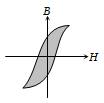(b)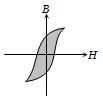(c)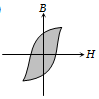(d)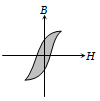Concept Questions :-

Curie law and hyterisis
High Yielding Test Series + Question Bank - NEET 2020

Difficulty Level:

The variation of magnetic susceptibility $\left(\chi \right)$ with temperature for a diamagnetic substance is best represented by

1.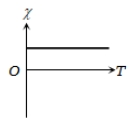2.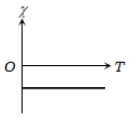3.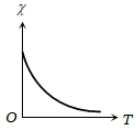4.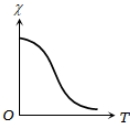Concept Questions :-

Magnetic materials
High Yielding Test Series + Question Bank - NEET 2020

Difficulty Level:

The variation of magnetic susceptibility $\left(\chi \right)$ with absolute temperature T for a ferromagnetic material is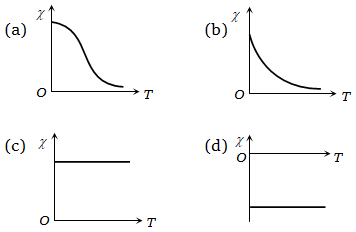Concept Questions :-

Curie law and hyterisis